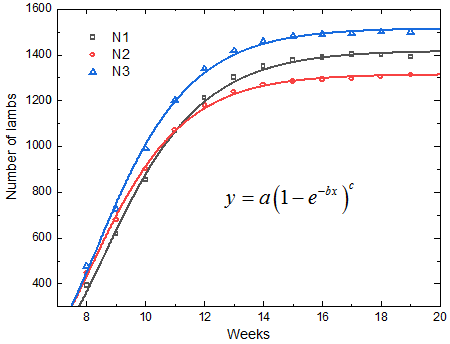# Estimate of population size after 20 weeks.

## Question:

Estimate of population size after 20 weeks.

Week N1 N2 N3 Lamb 1 Lamb 2 Lamb 3
8 392 449 476
9 616 679 726 1.568924414 1.513031954 1.553016087
10 852 902 990 1.384103165 1.328708274 1.364209078
11 1066 1072 1201 1.250768017 1.188363381 1.213335863
12 1211 1179 1339 1.136776624 1.0999052555 1.114815032
13 1300 1239 1418 1.072808078 1.050613686 1.058680776
14 1348 1270 1459 1.03748048 1.025017023 1.0291547
15 1374 1285 1480 1.018949754 1.012210773 1.1.014275675
16 1387 1293 1490 1.009491876 1.005923038 1.006939563
17 1400 1295 1492 1.009436426 1.001679153 1.001151106
18 1398 1304 1501 0.998571429 1.006949807 1.006032172
19 1390 1312 1499 0.994277539 1.006134969 0.998667555
Average Lamb 1.1346898 1.112594301 1.1236616
Overall Lamb 1.11116565 1.09353344 1.10199982
r 0.12635931 0.106694497 0.116592639
r 0.105409599 0.089414142 0.097126548

## Data fit

This is a problem that a rather ambiguous approach. Therefore, the best way to obtain information from the data provided is to fit the data. This is a statistical process by which a specific mathematical behavior is assigned to a group of experimental points.In our case, the experimental points are the number of lambs in each herd as a function of time. If there were much more information, a specific behavior could be assigned to the number of lambs, however in this case, this information is not available. Therefore a stretched asymptotic exponential equation of the type was chosen:

{eq}y=a(1-e^{-bx})^c {/eq}

For herd 1 we have:

{eq}N1(t)=1418\left ( 1-e^{-(0.52)t} \right )^{84}\\ N1(20\text{ weeks})=1418\left ( 1-e^{-(0.52)(20)} \right )^{84}\\ \therefore N1(20\text{ weeks})=1414\text{ lambs} {/eq}

For herd 2 we have:

{eq}N2(t)=1315\left ( 1-e^{-(0.56)t} \right )^{94}\\ N2(20\text{ weeks})=1315\left ( 1-e^{-(0.56)(20)} \right )^{94}\\ \therefore N2(20\text{ weeks})=1313\text{ lambs} {/eq}

For herd 3 we have:

{eq}N3(t)=1516\left ( 1-e^{-(0.54)t} \right )^{94}\\ N3(20\text{ weeks})=1516\left ( 1-e^{-(0.54)(20)} \right )^{94}\\ \therefore N3(20\text{ weeks})=1513\text{ lambs} {/eq}